# J. C. Sprott

Department of Physics, University of Wisconsin, Madison, WI 53706, USA
January 12, 2001
Last revised September 15, 2001

Perhaps the algebraically simplest examples of sinusoidally-driven conservative chaotic flows are of the form:

d2x/dt2 + f(x) = A sin wt

A variant of simulated annealing was used to find the value of w that maximizxes the Lyapunov exponent (base-e)  for A = 1 for various f(x) with the following results:

 Equation Lyapunov exponent d2x/dt2 + sin x = sin 0.50t 0.163 d2x/dt2 + x3 = sin 1.88t 0.097 d2x/dt2 + x5 = sin 2.19t 0.163 d2x/dt2 + x7 = sin 2.32t 0.198 d2x/dt2 + x9 = sin 2.58t 0.230 d2x/dt2 + x11 = sin 2.79t 0.242 d2x/dt2 + x3 - x = sin 1.87t 0.164 d2x/dt2 + x|x|-1/2 = sin 5.57t 0.123 d2x/dt2 + x|x| = sin 1.61t 0.051 d2x/dt2 + x|x|3 = sin 2.00t 0.139 d2x/dt2 + sinh x = sin 1.54t 0.014 d2x/dt2 + tanh x = sin 0.27t 0.009

All cases had initial conditions of dx/dt = x = 0.

Hans Gottlieb (private communication, 14 Dec 2000) has pointed out that the case with f(x) = x3 is especially interesting because it may be the simplest sinusoidally-driven chaotic system.  It is the dissipationless limit of the Ueda oscillator.  It has a single parameter w.  The plot below shows how the Lyapunov exponent for this system varies with w: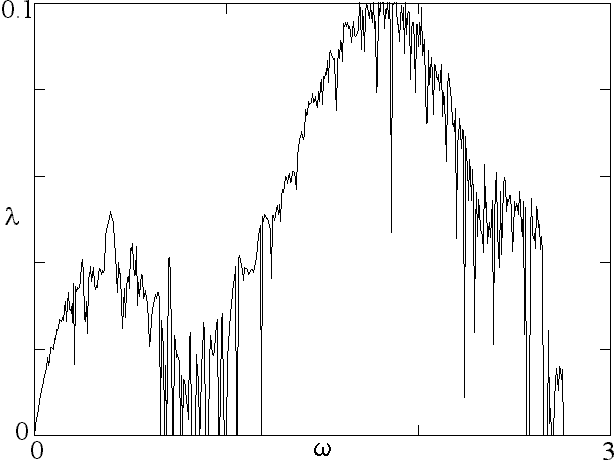A Poincare section in the x-dx/dt plane for wt mod 2pi = 0 is shown below: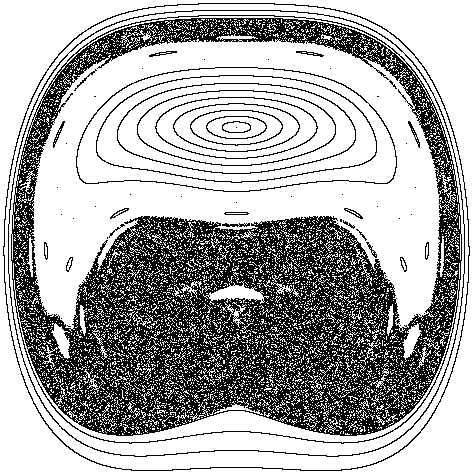More generally, the system

d2x/dt2 + x|x|p-1 = sin wt

has chaotic solutions for a range of p and w.  For example, the case p = 5 appears as follows: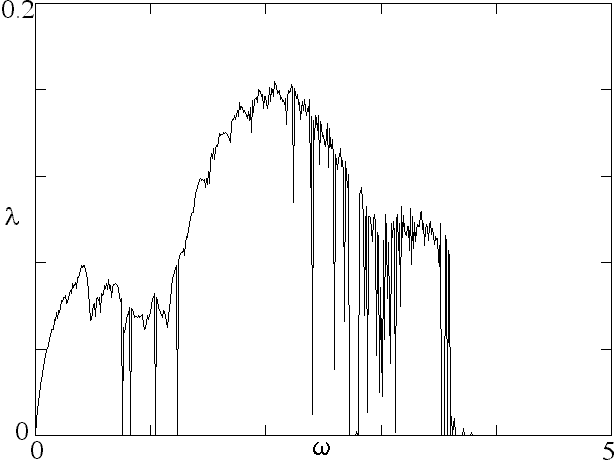The Lyapunov exponent for arbitrary p and w is shown in a gray scale below for initial conditions dx/dt = x = 0: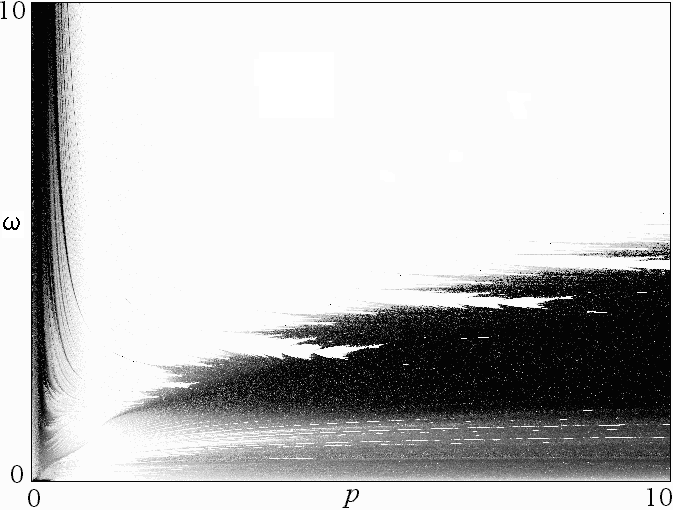Chaos also occurs in nonlinear oscillators driven with square waves rather than sine waves.  For example the system

d2x/dt2 + x3 = sgn(sin wt)

exhibits chaos over a wide range of w as shown below: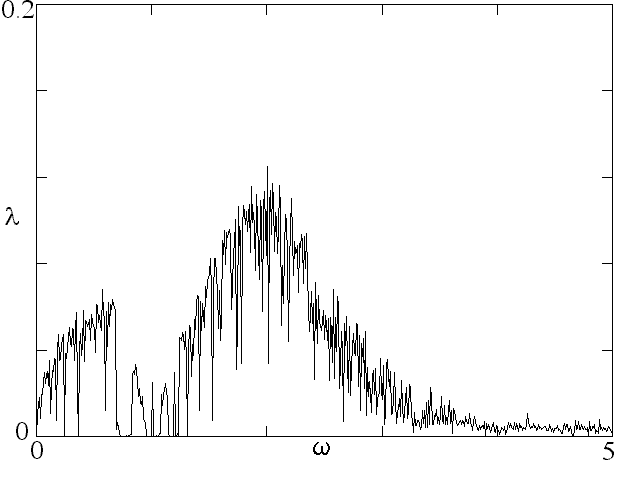This system has the virtue that it may be possible to find an analytic solution.

Ref: J. C. Sprott, Chaos and Time-Series Analysis (Oxford University Press, 2003), pp.192-193.